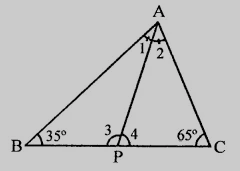# In $\triangle ABC, \angle B = 35^o, \angle C = 65^o$ and the bisector of $\angle BAC$ meets $BC$ in $P$. Arrange $AP, BP$ and $CP$ in descending order.

Given:

In $\triangle ABC, \angle B = 35^o, \angle C = 65^o$ and the bisector of $\angle BAC$ meets $BC$ in $P$.

To do:

We have to arrange $AP, BP$ and $CP$ in descending order.

Solution:We know that,

Sum of the angles in a triangle is $180^o$.

This implies,

$\angle A + \angle B + \angle C = 180^o$

$\angle A + 35^o + 65^o = 180^o$

$\angle A + 100^o = 180^o$

$\angle A =180^o - 100^o = 80^o$

$PA$ is a bisector of $\angle BAC$

This implies,

$\angle 1 = \angle 2 = \frac{80^o}{2} = 40^o$

In $\triangle ACP$,

$\angle ACP > \angle CAP$

This implies,

$\angle C > \angle 2$

Therefore,

$AP > CP$......…(i)

Similarly,

In $\triangle ABP$,

$\angle BAP > \angle ABP$

This implies,

$\angle 1 > \angle B$

Therefore,

$BP > AP$......…(ii)

From (i) and (ii), we get,

$BP > AP > CP$

Updated on: 10-Oct-2022

27 Views